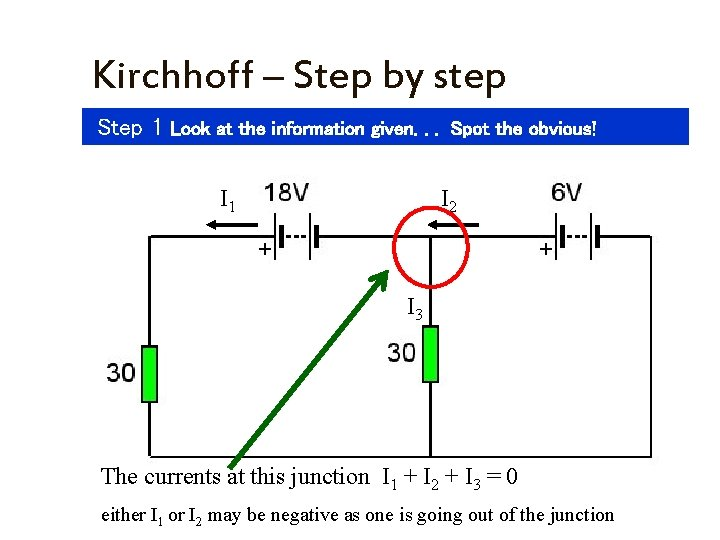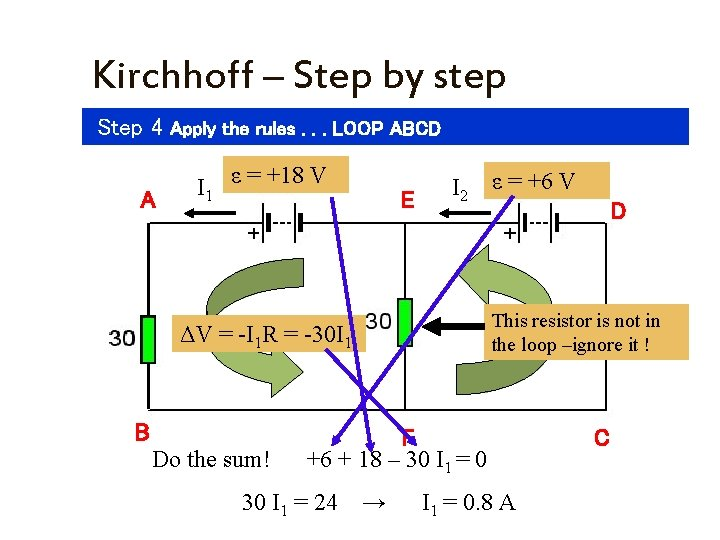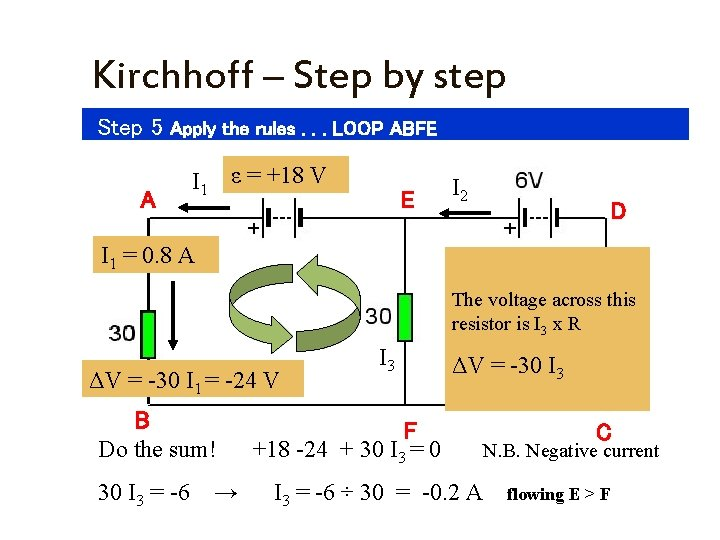# Kirchhoff Step by step Step 1 Look at

• Slides: 7Kirchhoff – Step by step Step 1 Look at the information given. . . Spot the obvious! I 1 I 2 The voltage across this resistor ΔV = IR = I 1 x 30 = 30 I 1Kirchhoff – Step by step Step 1 Look at the information given. . . Spot the obvious! I 1 I 2 I 3 The currents at this junction I 1 + I 2 + I 3 = 0 either I 1 or I 2 may be negative as one is going out of the junctionKirchhoff – Step by step Step 2 break the circuit into a set of Closed Loops & label them! A B I 1 E F I 2 D CKirchhoff – Step by step Step 3 Decide which way to go around the loop. . . A B I 1 E F I 2 D CKirchhoff – Step by step Step 4 Apply the rules. . . LOOP ABCD A I 1 ε = +18 V E I 2 ε = +6 V This resistor is not in the loop –ignore it ! ΔV = -I 1 R = -30 I 1 B Do the sum! F +6 + 18 – 30 I 1 = 0 30 I 1 = 24 D → I 1 = 0. 8 A CKirchhoff – Step by step Step 5 Apply the rules. . . LOOP ABFE A I 1 ε = +18 V E I 2 D I 1 = 0. 8 A The voltage across this resistor is I 3 x R ΔV = -30 I 1 = -24 V B Do the sum! 30 I 3 = -6 → I 3 ΔV = -30 I 3 F +18 -24 + 30 I 3 = -6 ÷ 30 = -0. 2 A C N. B. Negative current flowing E > FKirchhoff – Step by step Step 6 Apply the rules. . . Current at junction E I 1 A I 1 = 0. 8 A I 2 E I 3 = 0. 2 A B Do the sum F I 1 + I 2 + I 3 = 0 D Currents entering a junction are positive + I Currents leaving a junction negative - I C - 0. 8 – 0. 2 + I 3 = 0 → I 3 = 0. 8 + 0. 2 = 1. 0 A